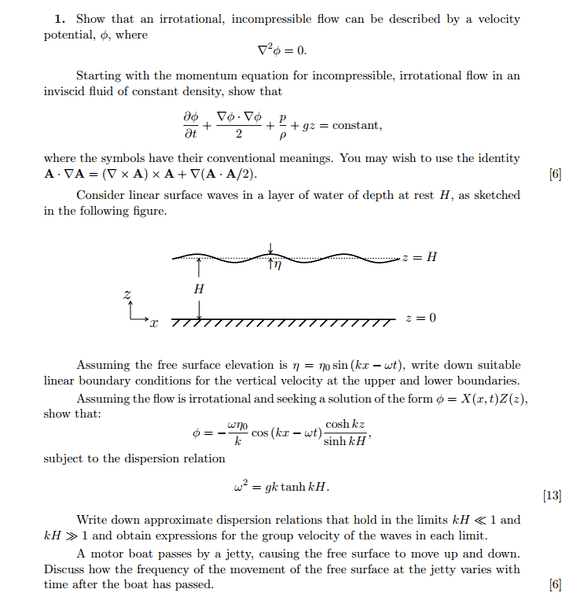# Navier Stokes Equation - Flow of waves

## Homework Statement

[/B]
(a) Show that for an incompressible flow the velocity potential satisfies ##\nabla^2 \phi = 0##. Show further the relation for the potential to be ## \frac{\partial \phi}{\partial t} + \frac{\nabla \phi \dot \nabla \phi}{2} + \frac{p}{\rho} + gz = const.##
(b)Write out the boundary conditions on velocity and show ##\phi\$## is of the form: ## \phi = -\frac{\omega n_0}{k} cos(kx-\omega t) \frac{cosh kz}{sinh kH}## subject to ## \omega^2 = gk \space tanh \space (kH)##
(c) How does the frequency of the waves vary after the boat is gone?## The Attempt at a Solution

Part (a)[/B]

For an irrotational flow, ## \nabla \cdot \vec u = 0## so this means that velocity can be written as gradient of some potential ## u = \nabla \phi##.

Therefore ## \nabla \cdot \vec u = \nabla^2 \phi = 0##.

Navier stokes equation is given by:
$$\frac{\partial \vec u}{\partial t} + (\vec u \cdot \nabla) \vec u + \frac{1}{\rho} \nabla p + g \vec k = \nu \nabla^2 \vec u$$

Using the identity and ## \nabla^2 \vec u = 0 ##, ## \nabla \times \vec u \times \vec u = 0## I get the answer.

Part (b)

The boundary condition is velocity at ##z=0## is zero (non-slip).

I don't think this question wants me to simply substitute the given form of ##\phi## to verify it is right. I am expected to derive it.

Substituting, I get
$$Z \frac{\partial X}{\partial t} + \frac{ (Z\nabla X + X \nabla Z) \cdot (Z\nabla X + X \nabla Z) }{2} + \frac{p}{\rho} + gz = const.$$

This leads to nowhere. Any idea how to proceed?